2017-03-09 13:24:23 zhangyibo123456789 阅读数 9518
• ###### MATLAB图像处理

全面系统的学习MATLAB在图像处理中的应用

19850 人正在学习 去看看 魏伟

# 浅析matlab图像滤波

1、首先我们要知道为什么要给图像滤波？

在尽量保留图像细节特征的条件下对目标图像的噪声进行抑制，是图像预处理中不可缺少的操作，其处理效果的好坏将直接影响到后续图像处理和分析的有效性和可靠性

2、什么是图像滤波？

由于成像系统、传输介质和记录设备等的不完善，数字图像在其形成、传输记录过程中往往会受到多种噪声的污染。另外，在图像处理的某些环节当输入的像对象并不如预想时也会在结果图像中引入噪声。这些噪声在图像上常表现为一引起较强视觉效果的孤立像素点或像素块。一般，噪声信号与要研究的对象不相关它以无用的信息形式出现，扰乱图像的可观测信息。
对于数字图像信号，噪声表为或大或小的极值，这些极值通过加减作用于图像像素的真实灰度值上，对图像造成亮、暗点干扰，极大降低了图像质量，影响图像复原、分割、特征提取、图像识别等后继工作的进行。
要构造一种有效抑制噪声的滤波器必须考虑两个基本问题：能有效地去除目标和背景中的噪声;同时，能很好地保护图像目标的形状、大小及特定的几何和拓扑结构特征

3、常见的滤波器有哪些？

也称均值滤波算法，主要思想为邻域平均法，即用几个像素灰度的平均值来代替每个像素的灰度。有效抑制加性噪声，但容易引起图像模糊，可以对其进行改进，主要避开对景物边缘的平滑处理。

中值滤波由Turky在1971年提出，最初用于时间序列分析，后来被用于图像处理，并在去噪复原中取得了较好的效果。中值滤波器是基于次序统计完成信号恢复的一种典型的非线性滤波器，其基本原理是把图像或序列中心点位置的值用该域的中值替代，具有运算简单、速度快、除噪效果好等优点，曾被认为是非线性滤波的代表。然而，一方面中值滤波因不具有平均作用，在滤除诸如高斯噪声时会严重损失信号的高频信息，使图像的边缘等细节模糊；另一方面中值滤波的滤波效果常受到噪声强度以及滤波窗口的大小和形状等因素的制约，为了使中值滤波器具有更好的细节保护特性及适应性，人们提出了许多中值滤波器的改进算法。
标准中值滤波算法的基本思想是将滤波窗口内的最大值和最小值均视为噪声，用滤波窗口内的中值代替窗口中心像素点的灰度，在一定程度上抑制了噪声。实际上在一定邻域范围内具有最大或最小灰度值这一特性的，除了噪声点，还包括图像中的边缘点、线性特征点等。中值滤波以此作为图像滤波依据，其滤波结果不可避免地会破坏图像的线段、锐角等信息。因此，要找到一种既能实现有效滤除噪声，又能完整保留图像细节的滤波机制，仅考虑噪声的灰度特性是难以实现的。

Wiener维纳滤波：使原始图像和其恢复图像之间的均方误差最小的复原方法，是一种自适应滤波器，根据局部方差来调整滤波器效果。对于去除高斯噪声效果明显。

随着数学各分支在理论和应用上的逐步深入，以数学形态学为代表的非线性滤波在保护图像边缘和细节方面取得了显著进展。形态学滤波器是近年来出现的一类重要的非线性滤波器，它由早期的二值形滤波器发展为后来的多值(灰度)形态滤波器，在形状识别、边缘检测、纹理分析、图像恢复和增强等领域了广泛的应用。
形态滤波方法充分利用形态学运算所具有的几何特征和良好的代数性质，主要采用态学开、闭运算进行滤波操作。从形态学基本原理可知，形态学的开运算会去掉图像上与结构元素的形态不相吻合的相对亮的分布结构，同时保留那些相吻合的部分;而闭运算则会填充那些图像上与结构元素不相吻合的相对暗的分布结构，同时保留那些相吻合的部分。因此他们都可以用来有效的提取特征和平滑像。值得注意地是，采用形态滤波器时，应根据不同的目的选择具有不同形状、大小和方向特性的结构元素。
此外，形态学开、闭运算都具有幂等性，这意味着一次滤波就己将所有特定于结构元素的噪声滤除千净，再次重复不会产生新的结果。这是一个经典方法(如线性卷积滤波、中值滤波)所不具备的性质。由于形态学运算是从图像的几何形态观点来进行图像处理的，因此这种优良的非线性滤波器能在滤波的同时，保持图像结构不被钝化。

4、图像去噪典的型算法及matlab实现

（1）、均值滤波matlab程序参考：
```I=imread('C:\Documents and Settings\Administrator\桌面\1.gif');%读取图像

J=imnoise(I,'gaussian',0,0.005);%加入均值为0，方差为0.005的高斯噪声

subplot(2,3,1);imshow(I);

title('原始图像');

subplot(2,3,2); imshow(J);

title('加入高斯噪声之后的图像');

%采用MATLAB中的函数filter2对受噪声干扰的图像进行均值滤波

K1=filter2(fspecial('average',3),J)/255; %模板尺寸为3

K2=filter2(fspecial('average',5),J)/255;% 模板尺寸为5

K3=filter2(fspecial('average',7),J)/255; %模板尺寸为7

K4= filter2(fspecial('average',9),J)/255; %模板尺寸为9

subplot(2,3,3);imshow(K1);

title('改进后的图像1');

subplot(2,3,4); imshow(K2);

title('改进后的图像2');

subplot(2,3,5);imshow(K3);

title('改进后的图像3');

subplot(2,3,6);imshow(K4);

title('改进后的图像4');```
（2）、二维自适应维纳滤波matlab程序参考：
```I=imread('C:\Documents and Settings\Administrator\桌面\1.gif'); %读取图像

J=imnoise(I,'gaussian',0,0.005); %加入均值为0，方差为0.005的高斯噪声

K2=wiener2(J,[3 3]); %对加噪图像进行二维自适应维纳滤波

K2=wiener2(J,[5 5]); %对加噪图像进行二维自适应维纳滤波

K2=wiener2(J,[7 7]); %对加噪图像进行二维自适应维纳滤波

K2=wiener2(J,[9 9]); %对加噪图像进行二维自适应维纳滤波

subplot(2,3,1);imshow(I);

title('原始图像');

subplot(2,3,2);imshow(J);

title('加噪图像');

subplot(2,3,3);imshow(K1);

title('恢复图像1');

subplot(2,3,4);imshow(K2);

title('恢复图像2');

subplot(2,3,5);imshow(K3);

title('恢复图像3');

subplot(2,3,6);imshow(K4);

title('恢复图像3');```

（3）、matlab图像处理形态学滤波教程详细参考：
http://blog.csdn.net/zhangyibo123456789/article/details/60955182

：均值滤波、中值滤波以及二维自适应维纳滤波的详细比较（带图）
http://www.cnblogs.com/xiangshancuizhu/archive/2011/01/04/1925276.html

matlab中图像处理滤波 相关内容

2019-12-06 14:59:55 LLeetion 阅读数 26
• ###### MATLAB图像处理

全面系统的学习MATLAB在图像处理中的应用

19850 人正在学习 去看看 魏伟

1.空域高斯滤波器

2.中值滤波

3.频率域高斯滤波

4.滤波器测试脚本

5.测试结果

# 1.空域高斯滤波器

``````function [OutImage,ConvAarray] = FilterGauss(InputImage,Size)
%================================================================
% 功能：单通道图像高斯滤波器
% 参数：InputImage为输入单通道图像，Size为模板尺寸
% 返回值：OutImage为InputImage同维数组
% 主要思路：利用卷积核，模板对称，利用相关等于卷积
% 备注：如果图像为多通道则需要重复调用
% 调用方法：OutImage = FilterGauss(InputImage)
% 日期：2019.12.02
% 作者：Leetion
[iLimit,jLimit] = size(InputImage);
OutImage = InputImage;
switch (Size)
case 3
ConvAarray =double((1/16)*[1 2 1;2 4 2;1 2 1]);
for yIndex = 2:iLimit-1
for xIndex = 2:jLimit-1
NeighbourArray = [InputImage(yIndex-1,xIndex-1) InputImage(yIndex-1,xIndex) InputImage(yIndex-1,xIndex+1);...
InputImage(yIndex,xIndex-1) InputImage(yIndex,xIndex) InputImage(yIndex,xIndex+1);...
InputImage(yIndex+1,xIndex-1) InputImage(yIndex+1,xIndex) InputImage(yIndex+1,xIndex+1)];
OutImage(yIndex,xIndex) = uint8(sum(sum(ConvAarray.*double(NeighbourArray))));
end
end
case 5
ConvAarray =double((1/90)*[1 2 4 2 1;2 4 8 4 2;4 8 16 8 4;2 4 8 4 2; 1 2 4 2 1]);
for yIndex = 3:iLimit-2
for xIndex = 3:jLimit-2
NeighbourArray = [InputImage(yIndex-2,xIndex-2) InputImage(yIndex-2,xIndex-1) InputImage(yIndex-2,xIndex) InputImage(yIndex-2,xIndex+1) InputImage(yIndex-2,xIndex+2);...
InputImage(yIndex-1,xIndex-2) InputImage(yIndex-1,xIndex-1) InputImage(yIndex-1,xIndex) InputImage(yIndex-1,xIndex+1) InputImage(yIndex-1,xIndex+2);...
InputImage(yIndex,xIndex-2) InputImage(yIndex,xIndex-1) InputImage(yIndex,xIndex) InputImage(yIndex,xIndex+1) InputImage(yIndex,xIndex+2);...
InputImage(yIndex+1,xIndex-2) InputImage(yIndex+1,xIndex-1) InputImage(yIndex+1,xIndex) InputImage(yIndex+1,xIndex+1) InputImage(yIndex+1,xIndex+2);...
InputImage(yIndex+2,xIndex-2) InputImage(yIndex+2,xIndex-1) InputImage(yIndex+2,xIndex) InputImage(yIndex+2,xIndex+1) InputImage(yIndex+2,xIndex+2)];
OutImage(yIndex,xIndex) = uint8(sum(sum(ConvAarray.*double(NeighbourArray))));
end
end
otherwise
warning("只支持三阶和五阶");
end
end

``````

# 2.中值滤波

``````function OutImage = FilterMiddle(InputImage,Size)
%================================================================
% 功能：单通道图像中值滤波器
% 参数：InputImage为输入单通道图像，Size为邻域尺寸
% 返回值：OutImage为InputImage同维数组
% 主要思路：求解邻域中值
% 备注：如果图像为多通道则需要重复调用
% 调用方法：OutImage = FilterMiddle(InputImage)
% 日期：2019.12.02
% 作者：Leetion
[iLimit,jLimit] = size(InputImage);
OutImage = InputImage;
if Size(1)==1 && Size(2)==3
flag = 1;
elseif Size(1)==1 && Size(2)==5
flag = 2;
elseif Size(1)==3 && Size(2)==1
flag = 3;
elseif Size(1)==5 && Size(2)==1
flag = 4;
elseif Size(1)==3 && Size(2)==3
flag = 5;
elseif Size(1)==5 && Size(2)==5
flag = 6;
else
warning("不支持的尺寸")
end
switch(flag)
case 1
for yIndex = 1:iLimit
for xIndex = 2:jLimit-1
NeighbourArray = [InputImage(yIndex,xIndex-1) InputImage(yIndex,xIndex) InputImage(yIndex,xIndex+1)];
OutImage(yIndex,xIndex) = median(NeighbourArray);
end
end
case 2
for yIndex = 1:iLimit
for xIndex = 3:jLimit-2
NeighbourArray = [InputImage(yIndex,xIndex-2) InputImage(yIndex,xIndex-1) InputImage(yIndex,xIndex) InputImage(yIndex,xIndex+1) InputImage(yIndex,xIndex+2)];
OutImage(yIndex,xIndex) = median(NeighbourArray);
end
end
case 3
for yIndex = 2:iLimit-1
for xIndex = 1:jLimit
NeighbourArray = [InputImage(yIndex-1,xIndex),InputImage(yIndex,xIndex),InputImage(yIndex+1,xIndex)];
OutImage(yIndex,xIndex) = median(NeighbourArray);
end
end
case 4
for yIndex = 3:iLimit-2
for xIndex = 1:jLimit
NeighbourArray = [InputImage(yIndex-2,xIndex) InputImage(yIndex-1,xIndex),InputImage(yIndex,xIndex),InputImage(yIndex+1,xIndex) InputImage(yIndex+2,xIndex)];
OutImage(yIndex,xIndex) = median(NeighbourArray);
end
end
case 5
for yIndex = 2:iLimit-1
for xIndex = 2:jLimit-1
NeighbourArray = [InputImage(yIndex-1,xIndex-1) InputImage(yIndex-1,xIndex) InputImage(yIndex-1,xIndex+1)...
InputImage(yIndex,xIndex-1) InputImage(yIndex,xIndex) InputImage(yIndex,xIndex+1)...
InputImage(yIndex+1,xIndex-1) InputImage(yIndex+1,xIndex) InputImage(yIndex+1,xIndex+1)];
OutImage(yIndex,xIndex) = median(NeighbourArray);
end
end
case 6
for yIndex = 3:iLimit-2
for xIndex = 3:jLimit-2
NeighbourArray = [InputImage(yIndex-2,xIndex-2) InputImage(yIndex-2,xIndex-1) InputImage(yIndex-2,xIndex) InputImage(yIndex-2,xIndex+1) InputImage(yIndex-2,xIndex+2)...
InputImage(yIndex-1,xIndex-2) InputImage(yIndex-1,xIndex-1) InputImage(yIndex-1,xIndex) InputImage(yIndex-1,xIndex+1) InputImage(yIndex-1,xIndex+2)...
InputImage(yIndex,xIndex-2) InputImage(yIndex,xIndex-1) InputImage(yIndex,xIndex) InputImage(yIndex,xIndex+1) InputImage(yIndex,xIndex+2)...
InputImage(yIndex+1,xIndex-2) InputImage(yIndex+1,xIndex-1) InputImage(yIndex+1,xIndex) InputImage(yIndex+1,xIndex+1) InputImage(yIndex+1,xIndex+2)...
InputImage(yIndex+2,xIndex-2) InputImage(yIndex+2,xIndex-1) InputImage(yIndex+2,xIndex) InputImage(yIndex+2,xIndex+1) InputImage(yIndex+2,xIndex+2)];
OutImage(yIndex,xIndex) = median(NeighbourArray);
end
end
end
end

``````

# 3.频率域高斯滤波

``````function OutImage = FreFilterGauss(InputImage,D0)
%================================================================
% 功能：频域高斯滤波
% 参数：InputImage为输入单通道图像,D0为截止频率
% 返回值：OutImage为输出图像
% 主要思路：FFT转换到频率域，频率域乘积高斯传函，再IFFT2到时域
% 备注：使用库函数计算FFT和IFFT
% 调用方法：OutImage = FreFilterGauss(InputImage,D0)
% 日期：2019.12.02
% 作者：Leetion
[iLimit,jLimit] = size(InputImage);
FreInputImage = fft2(InputImage);
for vIndex = 1:iLimit
for uIndex = 1:jLimit
Distance = sqrt((vIndex-(1+iLimit)/2)^2+(uIndex-(1+jLimit)/2)^2);
H = exp(-(Distance^2/(2*D0^2)));
FreOutImage(vIndex,uIndex) = H*FreInputImage(vIndex,uIndex);
end
end
OutImage = uint8(ifft2(FreOutImage));
end``````

# 4.滤波器测试脚本

``````%================================================================
% 功能：滤波器测试
% 主要思路：
% 备注：
% 日期：2019.11.29
% 作者：李习银
clc,clear
close all
figure(1)
subplot(2,3,1);
imshow(InputImage);
title("原图");
InputImage = imnoise(InputImage,'gaussian',0.001,0.001);
% InputImage = imnoise(InputImage,'salt & pepper');
subplot(2,3,2);
imshow(InputImage);
title("Gaussian Noise");
OutImage = FilterGauss(InputImage,3);
subplot(2,3,3);
imshow(OutImage);
title("Gaussian Filter");
subplot(2,3,4);
OutImage = FilterMiddle(InputImage,[5,5]);
imshow(OutImage);
title("Middle Filter");
subplot(2,3,5);
OutImage = FreFilterGauss(InputImage,1500);
imshow(OutImage);
title("FreGauss Filter");
figure(2)
subplot(2,3,1);
imshow(InputImage);
title("原图");
InputImage = imnoise(InputImage,'salt & pepper');
subplot(2,3,2);
imshow(InputImage);
title("Gaussian Noise");
OutImage = FilterGauss(InputImage,3);
subplot(2,3,3);
imshow(OutImage);
title("Gaussian Filter");
subplot(2,3,4);
OutImage = FilterMiddle(InputImage,[5,5]);
imshow(OutImage);
title("Middle Filter");
subplot(2,3,5);
OutImage = FreFilterGauss(InputImage,1500);
imshow(OutImage);
title("FreGauss Filter");
``````

# 5.测试结果

matlab中图像处理滤波 相关内容

2018-09-26 21:39:34 weixin_42664854 阅读数 231
• ###### MATLAB图像处理

全面系统的学习MATLAB在图像处理中的应用

19850 人正在学习 去看看 魏伟

## 中值滤波

``````I2=medfilt2(I1,[m,n])
``````

``````i=rgb2gray(imread('songshu.jpg'));
inoise=imnoise(i,'salt & pepper');
w1=[1 2 1;2 4 2;1 2 1]/16;
output1=imfilter(inoise,w1,'conv','replicate');
w2=[1 1 1;1 1 1;1 1 1]/9;
output2=imfilter(inoise,w2,'conv','replicate');
output3=medfilt2(inoise,[3,3]);
%高斯滤波
subplot(131),imshow(output1);
%均值滤波
subplot(132),imshow(output2);
%中值滤波
subplot(133),imshow(output3);
``````

## 双边滤波

``````I=imread('songshu.jpg');
I=double(I)/255;
w=5;
sigma=[3 0.1];
B=bilateral_filter(I,w,sigma);
subplot(144),imshow(B);
``````

matlab中图像处理滤波 相关内容

2019-03-14 10:11:12 qq_36758461 阅读数 1058
• ###### MATLAB图像处理

全面系统的学习MATLAB在图像处理中的应用

19850 人正在学习 去看看 魏伟

## 均值滤波

均值滤波也称为线性滤波，其采用的主要方法为邻域平均法。是典型的线性滤波算法，它是指在图像上对目标像素给一个模板，该模板包括了其周围的临近像素（以目标像素为中心的周围8个像素，构成一个滤波模板，即去掉目标像素本身），再用模板中的全体像素的平均值来代替原来像素值。

## 中值滤波

中值滤波法是一种非线性平滑技术，它将每一像素点的灰度值设置为该点某邻域窗口内的所有像素点灰度值的中值. 中值滤波对脉冲噪声有良好的滤除作用，特别是在滤除噪声的同时，能够保护信号的边缘，使之不被模糊。这些优良特性是线性滤波方法所不具有的。此外，中值滤波的算法比较简单，也易于用硬件实现。

## 运行结果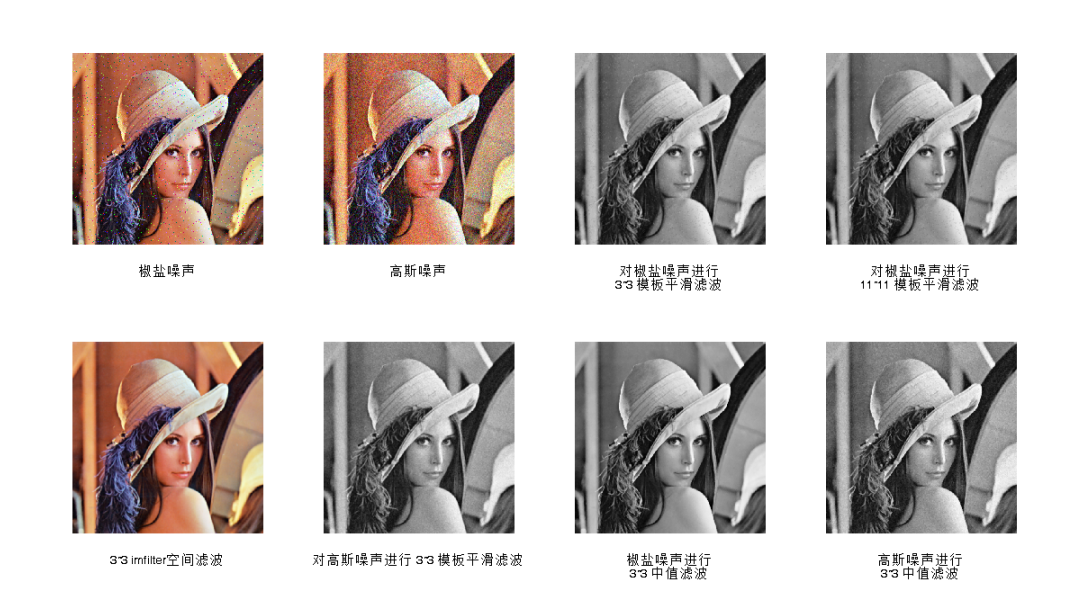## 程序代码

``````I = imread('G:\matlabEXCISIZE\execise\img\lena.png');
J1=imnoise(I,'salt & pepper',0.02); %加均值为0，方差为0.02的椒盐噪声
J2=imnoise(I,'gaussian',0.02); %加均值为0，方差为0.02的高斯噪声。
g1=rgb2gray(J1);
g2=rgb2gray(J2);
figure('units','normalized','position',[0 0 1 1]);
subplot(2,4,1),imshow(J1),xlabel('椒盐噪声'); %显示有椒盐噪声图像
subplot(2,4,2),imshow(J2),xlabel('高斯噪声'); %显示有高斯噪声图像

% %  邻域平均法  neighborhood averaging

% imfilter 可进行多维图像（RGB等）进行空间滤波，且可选参数较多
% filter2 / medfilt2 只能对二维图像（灰度图）进行空间滤波,
% 两个函数结果类型不一样，只需要在I1=filter2(h,I)后面加上I1=uint8(I1)进行类型转换，结果就是一样的。

K1 = filter2(fspecial('average',3),g1); % 对椒盐噪声进行 3*3 模板平滑滤波
K2 = filter2(fspecial('average',11),g1);
k3 = imfilter(I,fspecial('average',3),'replicate');
K4 = filter2(fspecial('average',3),g2); % 对高斯噪声进行 3*3 模板平滑滤波

subplot(2,4,3),imshow(uint8(K1)),xlabel({'对椒盐噪声进行';'3*3 模板平滑滤波'});
subplot(2,4,4),imshow(uint8(K1)),xlabel({'对椒盐噪声进行';'11*11 模板平滑滤波'});
subplot(2,4,5),imshow(k3),xlabel('3*3 imfilter空间滤波');
subplot(2,4,6),imshow(uint8(K4)),xlabel('对高斯噪声进行 3*3 模板平滑滤波');

% 中值滤波
I1= medfilt2(g1,[3,3]); % 对有椒盐噪声图像进行5×5方形窗口中值滤波
I2= medfilt2(g2,[3,3]); % 对有高斯噪声图像进行5×5方形窗口中值滤波

subplot(2,4,7),imshow(I1),xlabel({'椒盐噪声进行';'3*3 中值滤波'});
subplot(2,4,8),imshow(I2),xlabel({'高斯噪声进行';'3*3 中值滤波'});

``````

matlab中图像处理滤波 相关内容

2018-01-04 14:08:25 baidu_34971492 阅读数 36862
• ###### MATLAB图像处理

全面系统的学习MATLAB在图像处理中的应用

19850 人正在学习 去看看 魏伟

基于MATLAB图像处理的中值滤波、均值滤波以及高斯滤波的实现与对比

1.  背景知识

中值滤波法是一种非线性平滑技术，它将每一像素点的灰度值设置为该点某邻域窗口内的所有像素点灰度值的中值.

中值滤波是基于排序统计理论的一种能有效抑制噪声的非线性信号处理技术，中值滤波的基本原理是把数字图像或数字序列中一点的值用该点的一个邻域中各点值的中值代替，让周围的像素值接近的真实值，从而消除孤立的噪声点。

方法是用某种结构的二维滑动模板，将板内像素按照像素值的大小进行排序，生成单调上升（或下降）的为二维数据序列。二维中值滤波输出为g（x,y）=med{f(x-k,y-l),(k,l∈W)} ，其中，f(x,y)，g(x,y)分别为原始图像和处理后图像。W为二维模板，通常为3*3，5*5区域，也可以是不同的的形状，如线状，圆形，十字形，圆环形等。

 2 4 8 1 3 9 5 7 6

g（x,y）=med{f(x-k,y-l),(k,l∈W)}

g =med[2,4,8;1,3,9;5,7,6] = 5

中值滤波后的结果：

 5

均值滤波是典型的线性滤波算法，它是指在图像上对目标像素给一个模板，该模板包括了其周围的临近像素（以目标像素为中心的周围8个像素，构成一个滤波模板，即去掉目标像素本身），再用模板中的全体像素的平均值来代替原来像素值。

均值滤波也称为线性滤波，其采用的主要方法为邻域平均法。线性滤波的基本原理是用均值代替原图像中的各个像素值，即对待处理的当前像素点（x，y），选择一个模板，该模板由其近邻的若干像素组成，求模板中所有像素的均值，再把该均值赋予当前像素点（x，y），作为处理后图像在该点上的灰度g（x，y），即g（x，y）=1/m ∑f（x，y） m为该模板中包含当前像素在内的像素总个数。

均值滤波本身存在着固有的缺陷，即它不能很好地保护图像细节，在图像去噪的同时也破坏了图像的细节部分，从而使图像变得模糊，不能很好地去除噪声点。

 2 4 8 1 3 9 5 7 6

gxy=1/m ∑fxy

g = (1/8)*(2+4+8+1+9+5+7+6)= 5

均值滤波后的结果

 5

高斯滤波是一种线性平滑滤波，适用于消除高斯噪声，广泛应用于图像处理的减噪过程。通俗的讲，高斯滤波就是对整幅图像进行加权平均的过程，每一个像素点的值，都由其本身和邻域内的其他像素值经过加权平均后得到。高斯滤波的具体操作是：用一个模板（或称卷积、掩模）扫描图像中的每一个像素，用模板确定的邻域内像素的加权平均灰度值去替代模板中心像素点的值。

2.  MATLAB实现

源码：

%%-------------------------------------------------------------------------------------------

%% 2018/01/03

%% lee

%% 137194782@qq.com

%% 微信公众号：FPGA开源工作室

%%--------------------------------------------------------------------------------------------

clear all;

clc;

figure,imshow(M);                %显示原始图像

title('original');

gray = rgb2gray(M);

figure,imshow(gray);                         %显示灰度图像

title('gray');

P1 = imnoise(gray,'gaussian',0.02);     %加入高斯躁声

figure,imshow(P1);                        %加入高斯躁声后显示图像

title('gaussiannoise');

P2 = imnoise(gray,'salt& pepper',0.02); %加入椒盐躁声

figure,imshow(P2);                        %加入椒盐躁声后显示图像

title('salt& pepper noise');

g = medfilt2(P1);                       %对高斯躁声中值滤波

figure,imshow(g);

title('medfiltergaussian');

h = medfilt2(P2);                       %对椒盐躁声中值滤波

figure,imshow(h);

title('medfiltersalt & pepper noise');

a=[1 1 1                               %对高斯躁声算术均值滤波

1 11

1 11];

l=1/9*a;

k = conv2(double(P1),double(l));

figure,imshow(k,[]);

title('arithmeticfiltergaussian');

d = conv2(double(P2),double(l));           %对椒盐躁声算术均值滤波

figure,imshow(d,[]);

title('arithmeticfiltersalt & pepper noise');

sigma=8;%标准差大小

window=double(uint8(3*sigma)*2+1);%窗口大小一半为3*sigma

H=fspecial('gaussian',window, sigma);%fspecial('gaussian',hsize, sigma)产生滤波模板

img_gauss=imfilter(P1,H,'replicate'); %为了不出现黑边，使用参数'replicate'（输入图像的外部边界通过复制内部边界的值来扩展）

figure, imshow(img_gauss);

title('gaussianfilting gauss noise');

img_salt=imfilter(P2,H,'replicate');

figure, imshow(img_salt);

title('gaussianfilting salt pepper noise');

结果展示：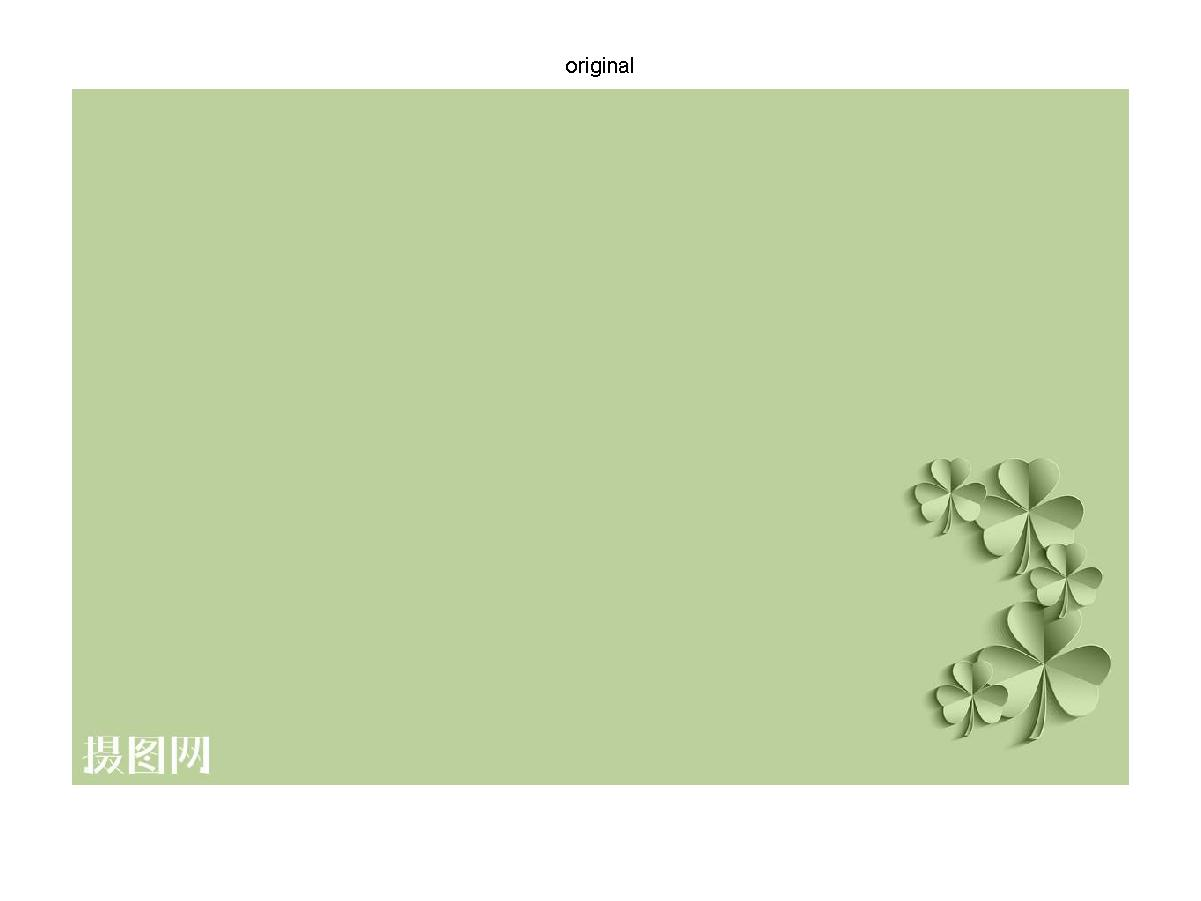原始图像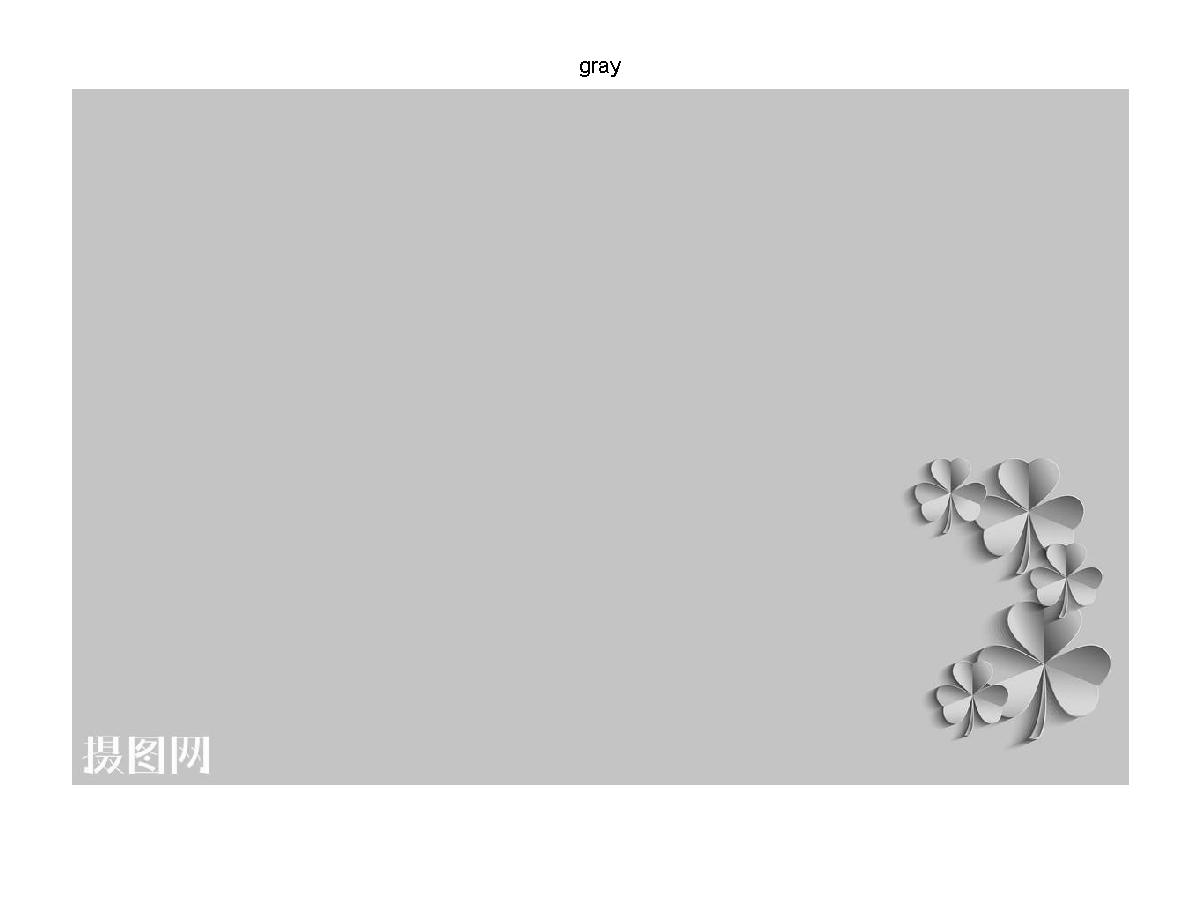灰度图像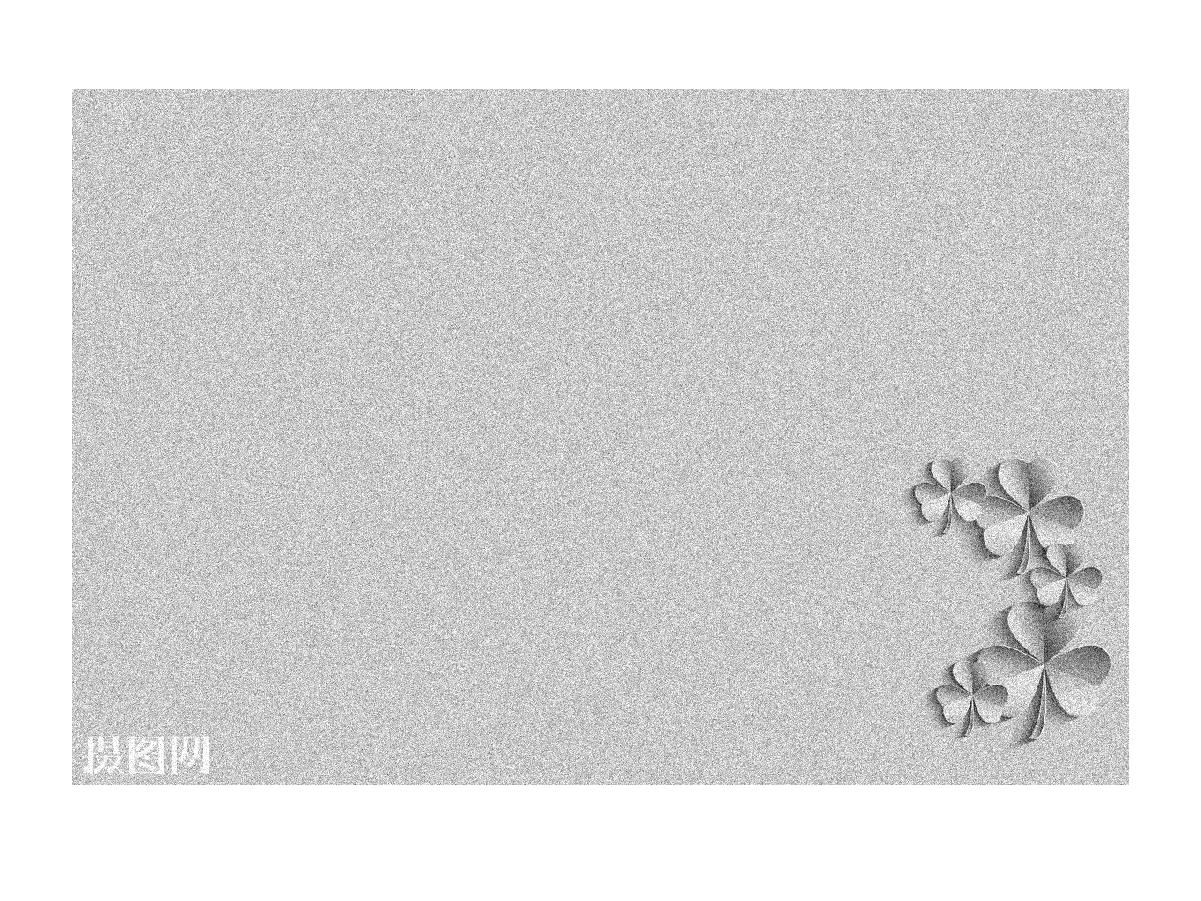加入高斯噪声的灰度图像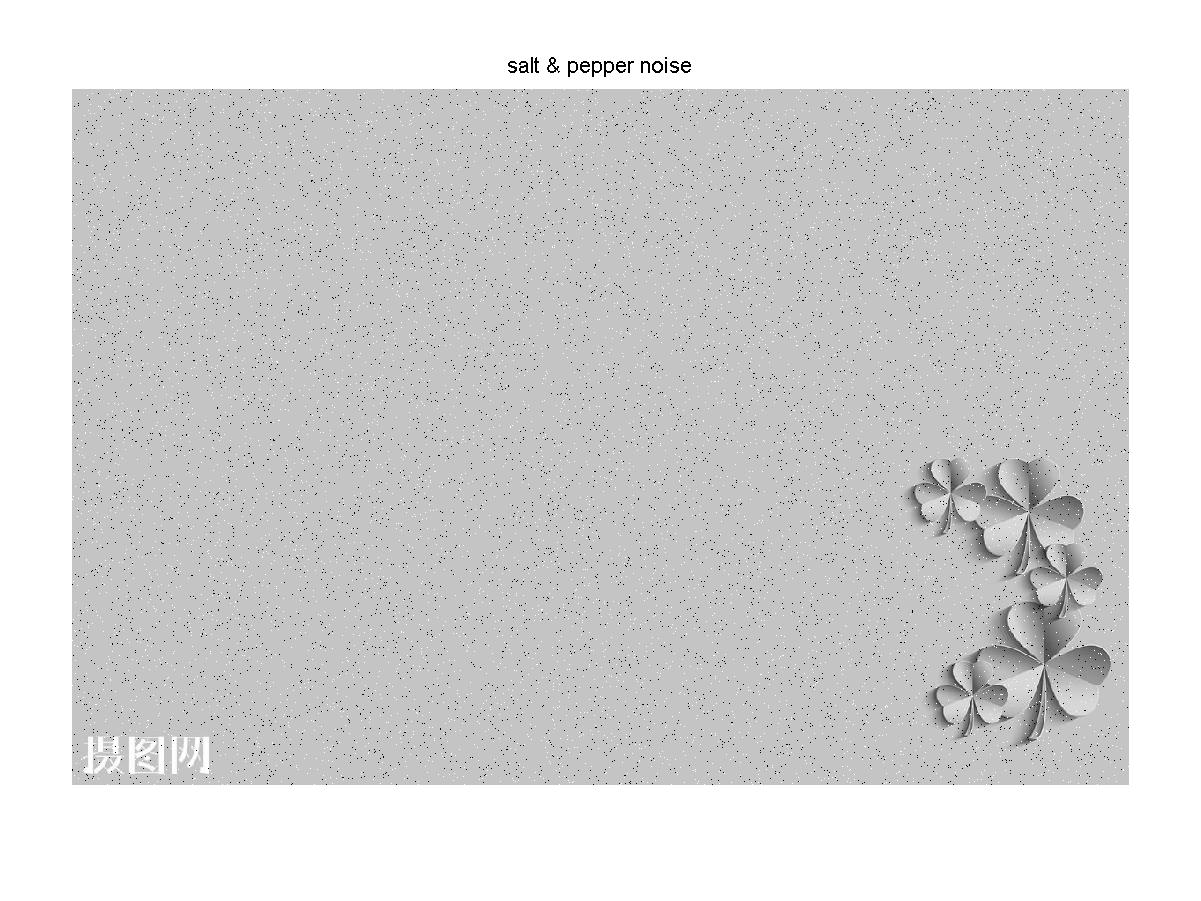加入椒盐噪声的灰度图像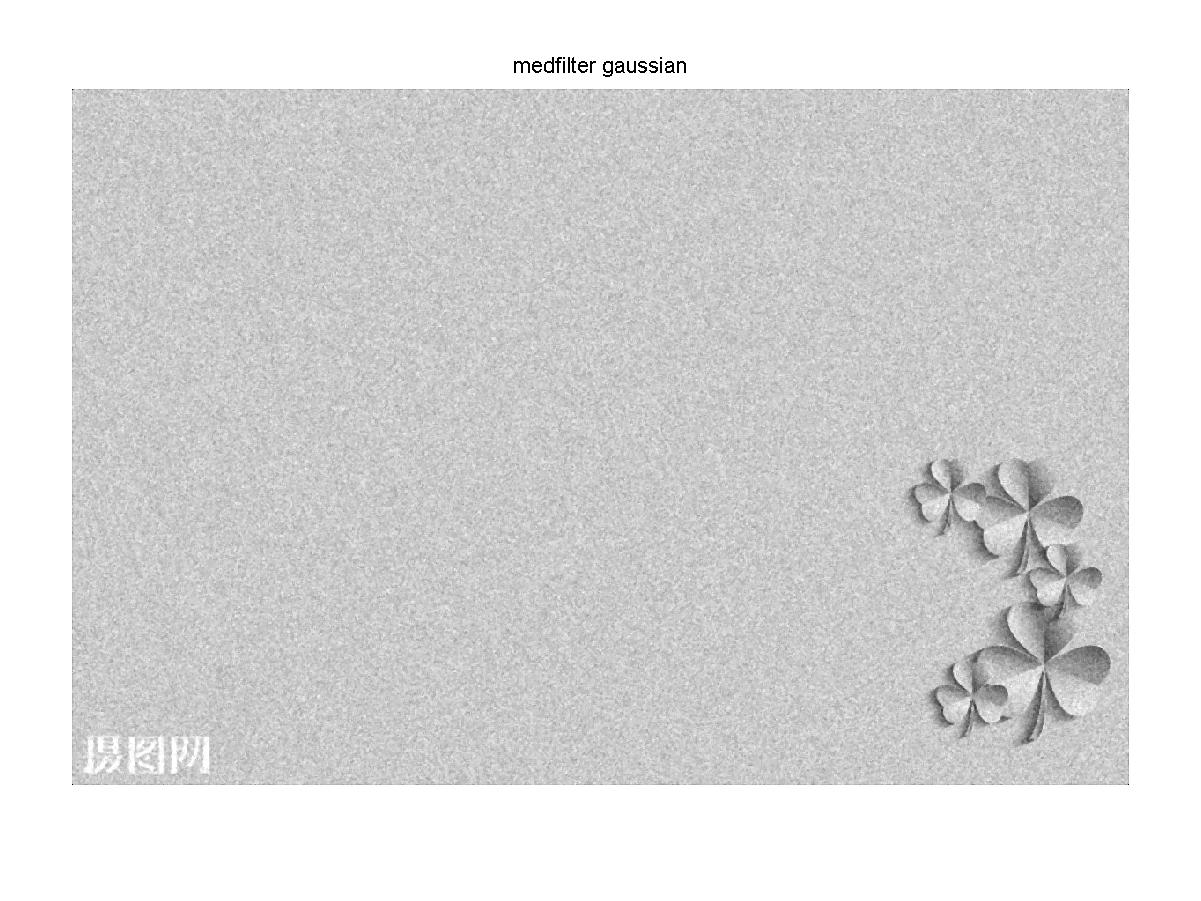经过中值滤波后的高斯噪声灰度图像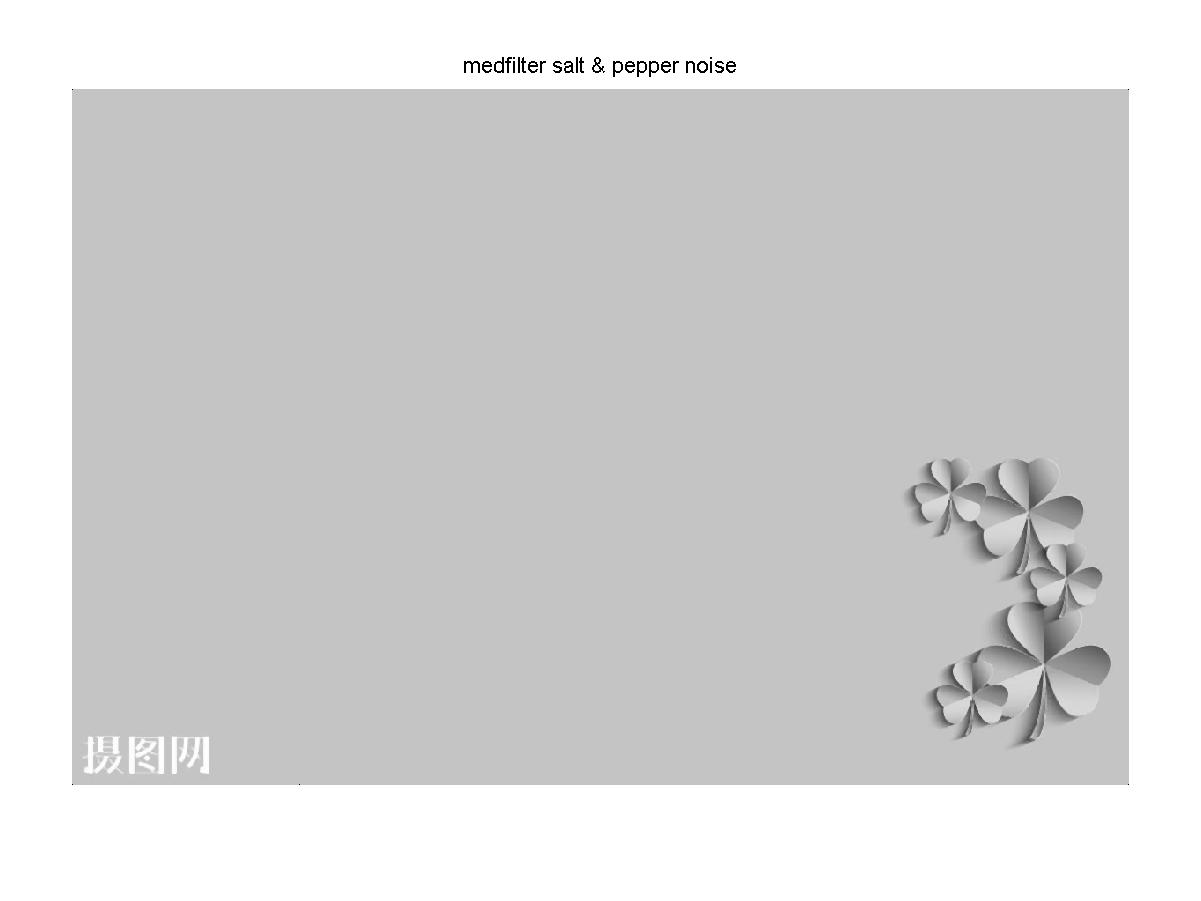经过中值滤波后的椒盐噪声灰度图像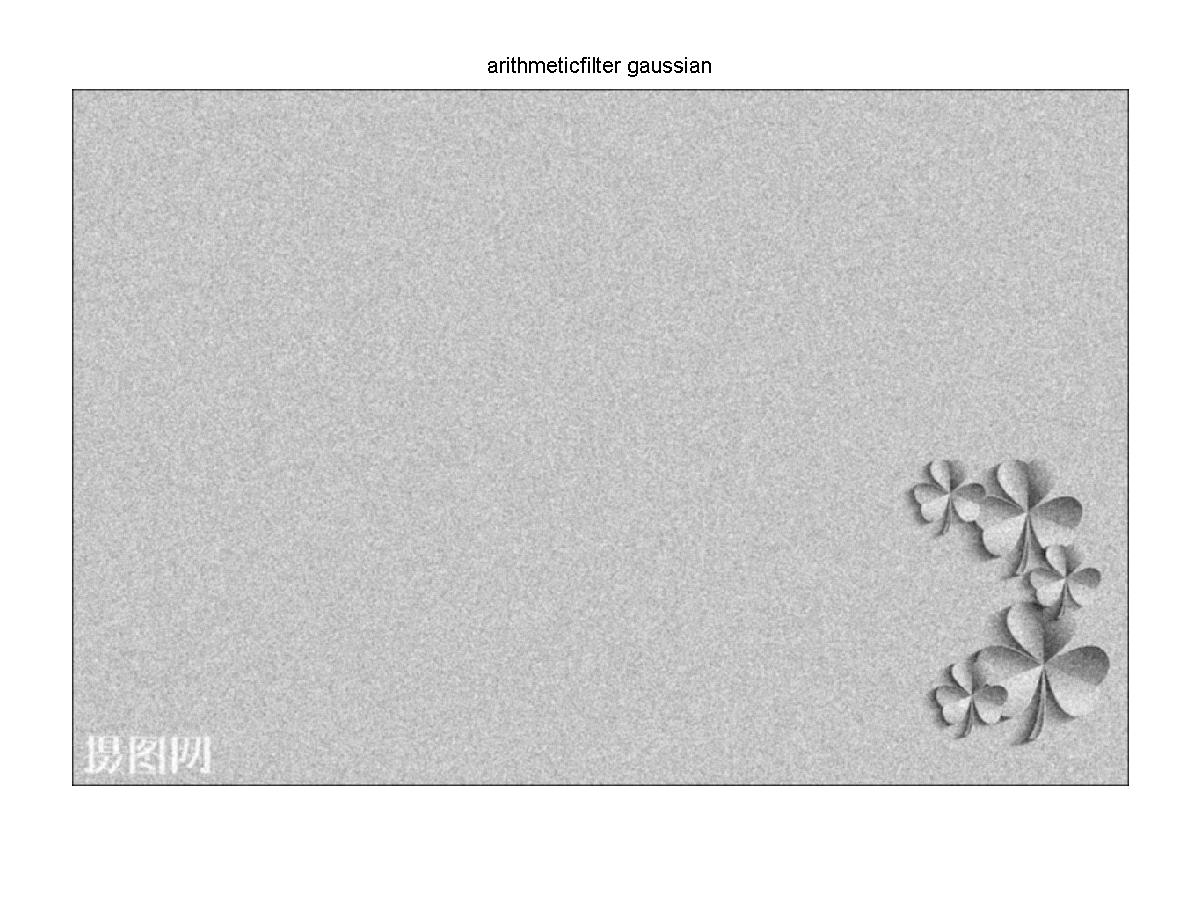经过均值滤波的高斯噪声灰度图像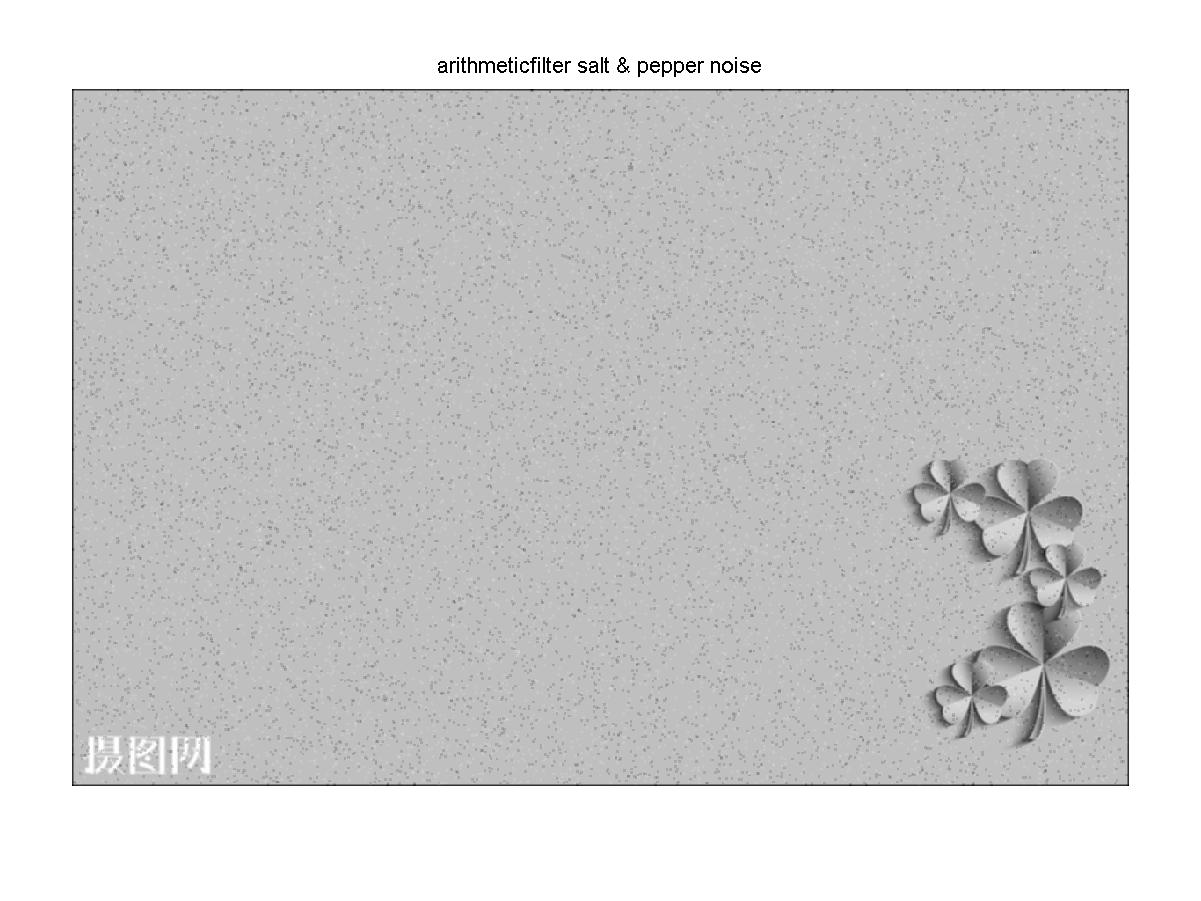经过均值滤波的椒盐噪声灰度图像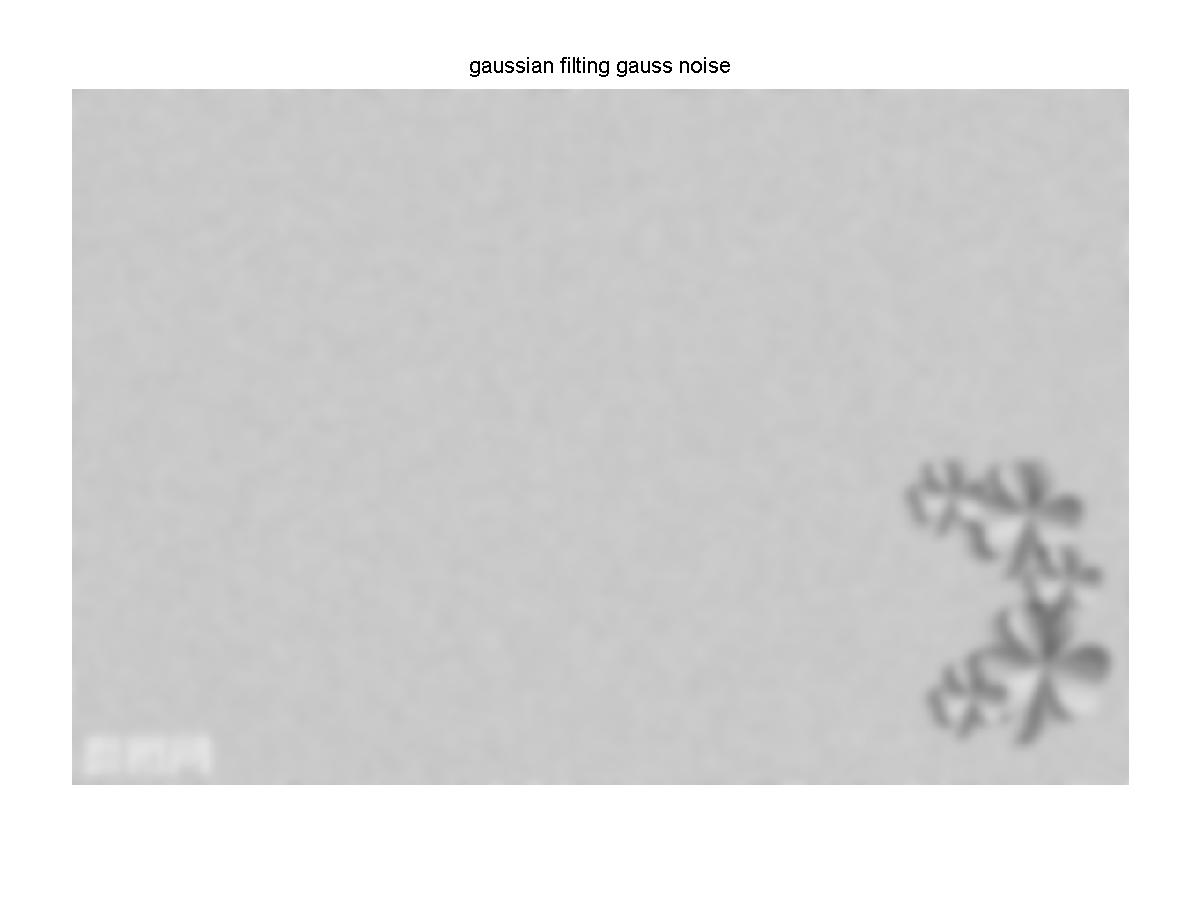经过高斯滤波的高斯噪声灰度图像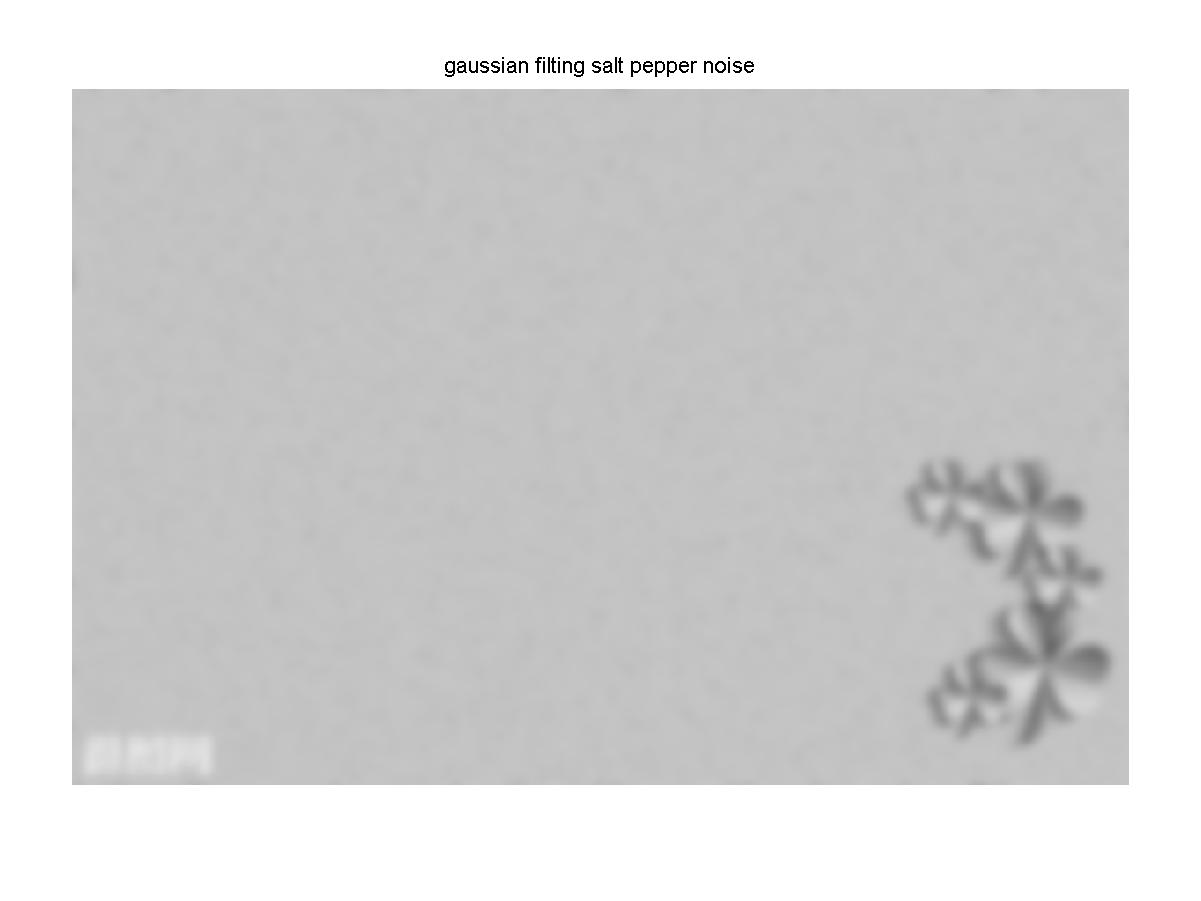经过高斯滤波的椒盐噪声的灰度图像

结果分析：图像经过中值滤波后，高斯噪声没有被完全去除，椒盐噪声几乎被完全去除效果较好。经过均值滤波后不管是高斯噪声还是椒盐噪声大部分都没有被去除，只是稍微模糊化。经过高斯滤波后，高斯噪声和椒盐噪声几乎被很大程度的模糊化，原图好像被加上了一层蒙版。

欢迎大家关注我的微信公众号FPGA开源工作室

FPGA自习学院将不断更新和总结FPGA相关学习资料，书籍，实例工程和视频。

欢迎大家加入FPGA自习学院，一起学习一起成长。matlab中图像处理滤波 相关内容

阅读数 14327

博文 来自： u014030117

阅读数 3293

阅读数 498

博文 来自： hhyqhh

阅读数 1097

博文 来自： weixin_44225182

阅读数 116

博文 来自： qq_40061319
没有更多推荐了，返回首页